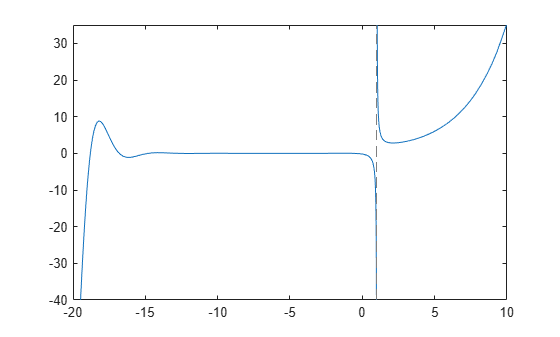# hurwitzZeta

Hurwitz zeta function

## Syntax

``Z = hurwitzZeta(s,a)``
``Z = hurwitzZeta(n,s,a)``

## Description

example

````Z = hurwitzZeta(s,a)` evaluates the Hurwitz zeta function for the numeric or symbolic inputs `s` and `a`. The Hurwitz zeta function is defined only if `s` is not 1 and `a` is neither 0 nor a negative integer.```

example

````Z = hurwitzZeta(n,s,a)` returns the `n`th derivative of `hurwitzZeta(s,a)` with respect to the variable `s`.```

## Examples

collapse all

Evaluate the Hurwitz zeta function with numeric input arguments.

`Z = hurwitzZeta(0,1)`
```Z = -0.5000 ```

Compute the symbolic output of `hurwitzZeta` by converting the inputs to symbolic numbers using `sym`.

`symZ = hurwitzZeta(sym([0 2]),1)`
```symZ =  $\left(\begin{array}{cc}-\frac{1}{2}& \frac{{\pi }^{2}}{6}\end{array}\right)$```

Use the `vpa` function to approximate symbolic results with the default 32 digits of precision.

`valZ = vpa(symZ)`
`valZ = $\left(\begin{array}{cc}-0.5& 1.644934066848226436472415166646\end{array}\right)$`

For certain parameter values, symbolic evaluation of the Hurwitz zeta function returns special values that are related to other symbolic functions.

For `a = 1`, the Hurwitz zeta function returns the Riemann zeta function `zeta`.

```syms s a; Z = hurwitzZeta(s,1)```
`Z = $\zeta \text{zeta}\left(s\right)$`

For `s = 2`, the Hurwitz zeta function returns the first derivative of the digamma function `psi`.

`Z = hurwitzZeta(2,a)`
`Z = ${\psi \text{psi}}^{\prime }\left(a\right)$`

For nonpositive integers `s`, the Hurwitz zeta function returns polynomials in terms of `a`.

`Z = hurwitzZeta(0,a)`
```Z =  $\frac{1}{2}-a$```
`Z = hurwitzZeta(-1,a)`
```Z =  $-\frac{{a}^{2}}{2}+\frac{a}{2}-\frac{1}{12}$```
`Z = hurwitzZeta(-2,a)`
```Z =  $-\frac{{a}^{3}}{3}+\frac{{a}^{2}}{2}-\frac{a}{6}$```

Find the first derivative of the Hurwitz zeta function with respect to the variable `s`.

```syms s a Z = hurwitzZeta(1,s,a)```
`Z = ${\zeta \text{hurwitzZeta}}^{\prime }\left(s,a\right)$`

Evaluate the first derivative at `s = 0` and `a = 1` by using the `subs` function.

`symZ = subs(Z,[s a],[0 1])`
```symZ =  $-\frac{\mathrm{log}\left(2\right)}{2}-\frac{\mathrm{log}\left(\pi \right)}{2}$```

Use the `diff` function to find the first derivative of the Hurwitz zeta function with respect to `a`.

`Z = diff(hurwitzZeta(s,a),a)`
`Z = $-s \zeta \text{hurwitzZeta}\left(s+1,a\right)$`

Plot the Hurwitz zeta function for `s` within the interval `[-20 10]`, given `a = 0.7`.

```fplot(@(s) hurwitzZeta(s,0.7),[-20 10]) axis([-20 10 -40 35]);```## Input Arguments

collapse all

Input, specified as a number, array, symbolic number, symbolic variable, symbolic function, symbolic expression, or symbolic array. The Hurwitz zeta function is defined only for values of `s` not equal to 1.

Data Types: `single` | `double` | `sym` | `symfun`
Complex Number Support: Yes

Input, specified as a number, array, symbolic number, symbolic variable, symbolic function, symbolic expression, or symbolic array. The Hurwitz zeta function is defined only for values of `a` not equal to 0 or a negative integer.

Data Types: `single` | `double` | `sym` | `symfun`
Complex Number Support: Yes

Order of derivative, specified as a nonnegative integer.

collapse all

### Hurwitz Zeta Function

The Hurwitz zeta function is defined by the formula

`$\zeta \left(s,a\right)=\sum _{k=0}^{\infty }\frac{1}{{\left(k+a\right)}^{s}}.$`

The summation series converges only when Re(s) > 1 and a is neither 0 nor a negative integer. Analytic continuation extends the definition of the function to the entire complex plane, except for a simple pole at s = 1.

## Tips

• Floating-point evaluation of the Hurwitz zeta function can be slow for complex arguments or high-precision numbers. To increase the computational speed, you can reduce the floating-point precision by using the `vpa` and `digits` functions. For more information, see Increase Speed by Reducing Precision.

• The Hurwitz zeta function is related to other special functions. For example, it can be expressed in terms of the polylogarithm Lis(z) and the gamma function Γ(z):

`$\zeta \left(1-s,a\right)=\frac{\Gamma \left(s\right)}{{\left(2\pi \right)}^{s}}\text{ }\text{\hspace{0.17em}}\left[{e}^{-i\pi s/2}\text{\hspace{0.17em}}{\text{Li}}_{s}\left({e}^{2\pi ia}\right)+{e}^{i\pi s/2}\text{\hspace{0.17em}}{\text{Li}}_{s}\left({e}^{-2\pi ia}\right)\right].$`

Here, Re(s) > 0 and Im(a) > 0, or Re(s) > 1 and Im(a) = 0.

 Olver, F. W. J., A. B. Olde Daalhuis, D. W. Lozier, B. I. Schneider, R. F. Boisvert, C. W. Clark, B. R. Miller, and B. V. Saunders, eds., Chapter 25. Zeta and Related Functions, NIST Digital Library of Mathematical Functions, Release 1.0.20, Sept. 15, 2018.